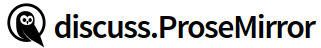# A question about the `liftTarget` function

I’m puzzled about the underlying reasoning behind choosing the depth that a NodeRange could be lifted to.

Assume we need to lift the second paragraph “cd” out of the block quote:

``````<doc>
<blockquote>
<p>ab</p>
<p>cd</p>
</blockquote>
</doc>
``````

We need to call `liftTarget(range)` where `range` covers the `<p>cd</p>` node.

``````export function liftTarget(range) {
let parent = range.parent
let content = parent.content.cutByIndex(range.startIndex, range.endIndex)
for (let depth = range.depth;; --depth) {
let node = range.\$from.node(depth)
let index = range.\$from.index(depth), endIndex = range.\$to.indexAfter(depth)
// line 21
if (depth < range.depth && node.canReplace(index, endIndex, content))
return depth
if (depth == 0 || node.type.spec.isolating || !canCut(node, index, endIndex)) break
}
}
``````

When the execution reaches line #21, the local variables are:

range.depth = 1

depth = 0

content = `<p>cd</p>`

node = the root “doc”

index = 0, endIndex = 1

Ofc, `node.canReplace(index, endIndex, content) ` would return `true`, but I wonder how could that alone indicate we can lift the `<p>cd</p>` to be a child of the root node?